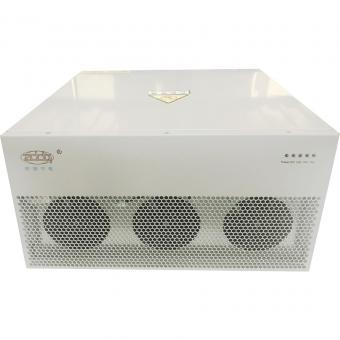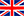English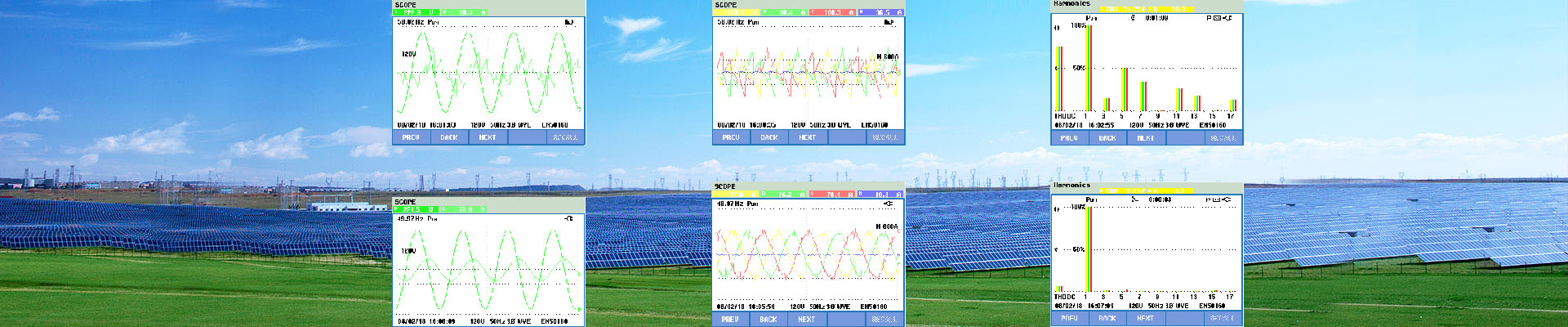###### Practical calculation of reactive power
Jan 08, 2018

Please refer to the following calculation examples

 Type of circuit Apparent power S (kVA) Active power P (kW) Reactive power Q (kVAr) Single phase (Ph + N) S = V x I P = V x I x cos φ Q = V x I x sin φ Single phase (Ph + Ph) S = U x I P = U x I x cos φ Q = U x I x sin φ Example:                                   5 kw load Cos φ= 0.5 10 kVA 5 Kw 8.7 kVAr Three-phase (3Ph or 3Ph+N) S = √3 x U x I P = √3 x U x I x cos φ Q = √3 x U x I x sin φ Example of Motor with               Pn = 51kW cos φ = 0.86                         efficiency = 0.91 65 kVA 56 kW 33 kVAr

Calculations in the three-phase example were as follows:
Pn = power supplied to the rotary axis = 51 kW
P = active consumed power = Pn/ρ = 56 kW
S = apparent power = P/cos φ = P/0.86 = 65 kVA
Hence:
Q = √(S² - P²) = √(65² - 56²) = 33 kVAr
The average power factor values for various loads are given below.

Hot Products
•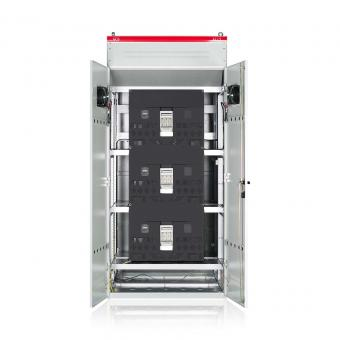#### Capacitor banks and harmonic filters. Low voltage

•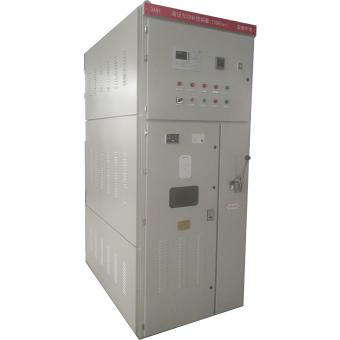#### 3.3KV Local reactive power compensation for motors,Arc Furnace

•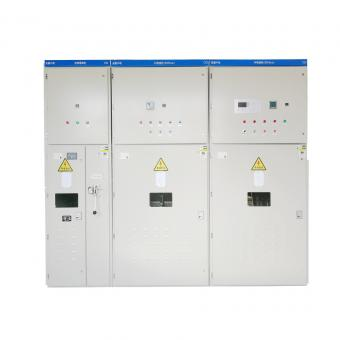#### Medium voltage Automatic Capacitor banks with harmonic filters

•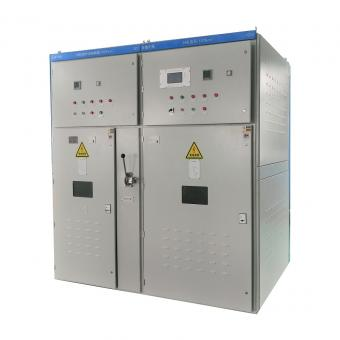#### High voltage Automatic Power Factor improvement plant for Motors VFDs

•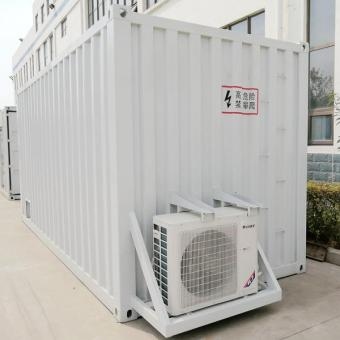#### High voltage static var generator HV SVG Statcom outdoor container

•#### Medium voltage outdoor Static Synchronous Compensator(STATCOM)

•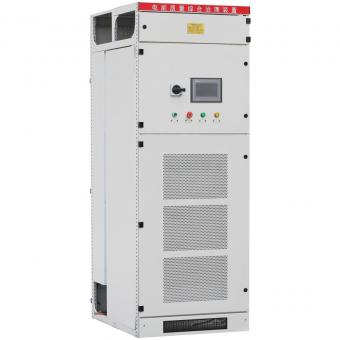•